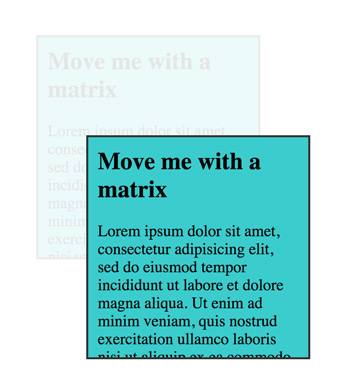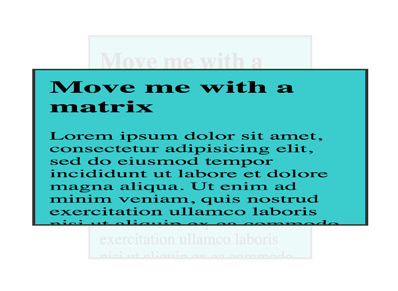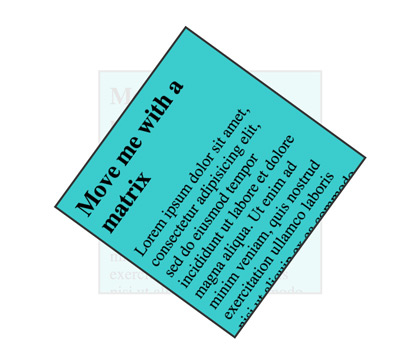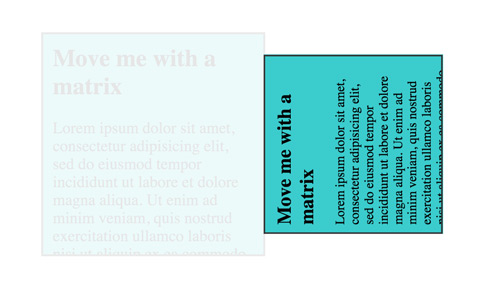/DOM

# WebGL API: Matrix math for the web

Matrices can be used to represent transformations of objects in space, and are an important tool to use in visualizations on the Web. This article explores how to create matrices and use them with CSS3 transforms and the `matrix3d` transform type.

While this article uses CSS3 for the ease of explanations, matrices are a core concept used by many different technologies including WebGL and shaders. This article is also available as an MDN content kit. The live examples use a collection of utility functions availabile under a global object named "MDN".

## What is a transformation matrix?

There are many types of matrices, but the ones we are interested in are the 3D transformation matrices. These matrices consist of a set of 16 values arranged in a 4x4 grid. In JavaScript, it is easy to represent a matrix as an array. The typical starting point is to show the identity matrix. When this matrix is multiplied against another point or matrix then the result will be identical.

```var identityMatrix = [
1, 0, 0, 0,
0, 1, 0, 0,
0, 0, 1, 0,
0, 0, 0, 1
];
```

Speaking of multiplication, what does this operation look like in a matrix? The easiest example is to show the multiplication of a single point. You may notice that a 3D point doesn't quite match up with a 4x4 matrix, so a fourth dimension W is added. For a typical position, setting this value to 1 will make the math work out. The W component has some additional uses that are out of scope for this article. Check out the WebGL model view projection article for a look into how it comes in handy.

Notice how the matrix and points line up:

```[1, 0, 0, 0,
0, 1, 0, 0,
0, 0, 1, 0,
0, 0, 0, 1]

[4, 3, 2, 1]
```

### Defining a multiplication function

In our example code we have defined a multiplication function — `multiplyMatrixAndPoint()`:

```function multiplyMatrixAndPoint(matrix, point) {

//Give a simple variable name to each part of the matrix, a column and row number
var c0r0 = matrix[ 0], c1r0 = matrix[ 1], c2r0 = matrix[ 2], c3r0 = matrix[ 3];
var c0r1 = matrix[ 4], c1r1 = matrix[ 5], c2r1 = matrix[ 6], c3r1 = matrix[ 7];
var c0r2 = matrix[ 8], c1r2 = matrix[ 9], c2r2 = matrix, c3r2 = matrix;
var c0r3 = matrix, c1r3 = matrix, c2r3 = matrix, c3r3 = matrix;

//Now set some simple names for the point
var x = point;
var y = point;
var z = point;
var w = point;

//Multiply the point against each part of the 1st column, then add together
var resultX = (x * c0r0) + (y * c0r1) + (z * c0r2) + (w * c0r3);

//Multiply the point against each part of the 2nd column, then add together
var resultY = (x * c1r0) + (y * c1r1) + (z * c1r2) + (w * c1r3);

//Multiply the point against each part of the 3rd column, then add together
var resultZ = (x * c2r0) + (y * c2r1) + (z * c2r2) + (w * c2r3);

//Multiply the point against each part of the 4th column, then add together
var resultW = (x * c3r0) + (y * c3r1) + (z * c3r2) + (w * c3r3);

return [resultX, resultY, resultZ, resultW];
}
```

Now using the function above we can multiply a point by the matrix. Using the identity matrix it should return the exact same value:

```// sets identityResult to [4,3,2,1]
var identityResult = multiplyMatrixAndPoint(identityMatrix, [4, 3, 2, 1]);
```

Returning the same point is not very useful, but there are other types of matrices that can perform helpful operations on points. The next sections will demonstrate some of these matrices.

### Multiplying two matrices

In addition to multiplying a matrix and a point together, you can also multiply two matrices together. The function from above can be re-used to help out in this process:

```function multiplyMatrices(matrixA, matrixB) {

// Slice the second matrix up into columns
var column0 = [matrixB, matrixB, matrixB, matrixB];
var column1 = [matrixB, matrixB, matrixB, matrixB];
var column2 = [matrixB, matrixB, matrixB, matrixB];
var column3 = [matrixB, matrixB, matrixB, matrixB];

// Multiply each column by the matrix
var result0 = multiplyMatrixAndPoint(matrixA, column0);
var result1 = multiplyMatrixAndPoint(matrixA, column1);
var result2 = multiplyMatrixAndPoint(matrixA, column2);
var result3 = multiplyMatrixAndPoint(matrixA, column3);

// Turn the result columns back into a single matrix
return [
result0, result1, result2, result3,
result0, result1, result2, result3,
result0, result1, result2, result3,
result0, result1, result2, result3
];
}
```

### Usage

Let's look at this function in action:

```var someMatrix = [
4, 0, 0, 0,
0, 3, 0, 0,
0, 0, 5, 0,
4, 8, 4, 1
]

var identityMatrix = [
1, 0, 0, 0,
0, 1, 0, 0,
0, 0, 1, 0,
0, 0, 0, 1
];

// Returns a new array equivalent to someMatrix
var someMatrixResult = multiplyMatrices(identityMatrix, someMatrix);
```

Important: These matrix functions are written for clarity of explanation, not for speed or memory management. These functions create a lot of new arrays, which can be particularly expensive for real time operations due to garbage collection. In real production code it would be best to use optimized functions. glMatrix is an example of a library that has a large focus on speed and performance. The focus in the glMatrix library is to have target arrays that are allocated before the update loop.

## Translation matrix

A translation matrix is based off the identity matrix. It moves the object in one of 3 directions, x, y, or z. The easiest way to think of a translation is like picking up a coffee cup. The coffee cup must be kept upright and oriented the same way so that no coffee is spilled. It can move up in the air off the table and around the air in space.

Now the coffee can't actually be drank with only a translation matrix because the cup cannot be tilted. In a later section, a new matrix will be discussed that will be able to handle that task.

```var x = 50;
var y = 100;
var z = 0;

var translationMatrix = [
1,    0,    0,   0,
0,    1,    0,   0,
0,    0,    1,   0,
x,    y,    z,   1
];
```

## Manipulating the DOM with a matrix

A really easy way to start using a matrix is to use the CSS3 `matrix3d` transform. First we'll set up a simple `<div>` with some content. The style is not shown. But it is set to a fixed width and height and centered on the page. The `<div>` has a transition set for the transform so that matrix is animated in making it easy to see what is being done.

```<div id='move-me' class='transformable'>
<h2>Move me with a matrix</h2>
<p>Lorem ipsum dolor sit amet, consectetur adipisicing elit...</p>
</div>
```

Finally for each of the examples we will generate a 4x4 matrix, then update the `<div>`'s style to have a transform applied to it, set to a `matrix3d`. Bear in mind that even though the matrix is made up of 4 rows and 4 columns, it collapses into a single line of 16 values.

```// Create the matrix3d style property from a matrix array
function matrixArrayToCssMatrix(array) {
return 'matrix3d(' + array.join(',') + ')';
}

// Grab the DOM element
var moveMe = document.getElementById('move-me');

// Returns a result like: "matrix3d(1, 0, 0, 0, 0, 1, 0, 0, 0, 0, 1, 0, 50, 100, 0, 1);"
var matrix3dRule = matrixArrayToCssMatrix(translationMatrix);

// Set the transform
moveMe.style.transform = matrix3dRule;
```

View on JSFiddle## Scale matrix

A scale matrix makes something larger or smaller in one of 3 dimension: width, height, and depth. In typical (cartesian) coordinates this would be stretching and shrinking in x, y, and z.

```var w = 1.5; // width  (x)
var h = 0.7; // height (y)
var d = 1;   // depth  (z)

var scaleMatrix = [
w,    0,    0,   0,
0,    h,    0,   0,
0,    0,    d,   0,
0,    0,    0,   1
];
```

View on JSFiddle## Rotation matrix

Rotation matrices start looking a little bit more complicated than scaling and transform matrices. They use trigonometric functions to perform the rotation. While this section won't break the steps down into exhaustive detail, take this example for illustration.

```// Manually rotating a point about the origin without matrices
var point = [10, 2];

// Calculate the distance from the origin
var distance = Math.sqrt(point * point + point * point);

// 60 degrees
var rotationInRadians = Math.PI / 3;

var transformedPoint = [
];
```

It is possible to encode these type of steps into a matrix, and do it each of the x, y, and z dimensions. Below is the representation of a rotation about the X axis:

```var sin = Math.sin;
var cos = Math.cos;

// NOTE: There is no perspective in these transformations, so a rotation
//       at this point will only appear to only shrink the div

var a = Math.PI * 0.3; //Rotation amount

// Rotate around Z axis
var rotateZMatrix = [
cos(a), -sin(a),    0,    0,
sin(a),  cos(a),    0,    0,
0,       0,    1,    0,
0,       0,    0,    1
];
```

View on JSFiddleHere are a set of functions that return the rotation matrices. One big note is that there is no perspective applied, so it might not feel very 3D yet. The flatness is equivalent to when a camera zooms in really close onto an object in the distance — the sense of perspective disappears.

```function rotateAroundXAxis(a) {

return [
1,       0,        0,     0,
0,  cos(a),  -sin(a),     0,
0,  sin(a),   cos(a),     0,
0,       0,        0,     1
];
}

function rotateAroundYAxis(a) {

return [
cos(a),   0, sin(a),   0,
0,   1,      0,   0,
-sin(a),   0, cos(a),   0,
0,   0,      0,   1
];
}

function rotateAroundZAxis(a) {

return [
cos(a), -sin(a),    0,    0,
sin(a),  cos(a),    0,    0,
0,       0,    1,    0,
0,       0,    0,    1
];
}
```

View on JSFiddle

## Matrix Composition

The real power of matrices come from matrix composition. When matrices of a certain class are multiplied together they preserve the history of the transformations and are reversible. This means that if a translation, rotation, and scale matrix are all combined together, when the order of the matrices is reversed and re-applied then the original points are returned.

The order that matrices are multiplied in matters. When multiplying numbers a * b = c, and b * a = c are both true. For example 3 * 4 = 12, and 4 * 3 = 12. In math these numbers would be described as commutative. Matrices are not guaranteed to be the same if the order is switched, so matrices are non-commutative.

Another mind-bender is that matrix multiplication in WebGL and CSS3 needs to happen in the reverse order that the operations intuitively happen. For instance, to scale something down by 80%, move it down 200 pixels, and then rotate about the origin 90 degrees would look something like the following in pseudo-code.

```  transformation = rotate * translate * scale
```

### Composing multiple transformations

The function that we will be using is part of the utility functions. It takes an array of matrices and multiplies them together. In WebGL shader code, this is built into the language and the * operator can be used. Additionally this example uses a scale and translate function which return matrices as defined above.

```var transformMatrix = MDN.multiplyArrayOfMatrices([
rotateAroundZAxis(Math.PI * 0.5),    // Step 3: rotate around 90 degrees
translate(0, 200, 0),                // Step 2: move down 100 pixels
scale(0.8, 0.8, 0.8),                // Step 1: scale down
]);

```

View on JSFiddleFinally a fun step to show how matrices work is to reverse the steps to bring the matrix back to the original identity matrix.

```var transformMatrix = MDN.multiplyArrayOfMatrices([
scale(1.25, 1.25, 1.25),             // Step 6: scale back up
translate(0, -200, 0),               // Step 5: move back up
rotateAroundZAxis(-Math.PI * 0.5),   // Step 4: rotate back
rotateAroundZAxis(Math.PI * 0.5),    // Step 3: rotate around 90 degrees
translate(0, 200, 0),                // Step 2: move down 100 pixels
scale(0.8, 0.8, 0.8),                // Step 1: scale down
]);
```

## Why matrices are important

Matrices are important because they comprise a small set of numbers that can describe a wide range of transformations in space. They can easily be shared around in programs. Different coordinate spaces can be described with matrices, and some matrix multiplication will move one set of data from one coordinate space to another coordinate space. Matrices effectively remember every part of the previous transforms that were used to generate them.

For uses in WebGL, the graphics card is particularly good at multiplying a large number of points in space by matrices. Different operations like positioning points, calculating lighting, and posing animated characters all rely on this fundamental tool.

© 2005–2018 Mozilla Developer Network and individual contributors.